HOME COURSES PREVIEW REVIEW ABOUT CONTACTToll-Free Info & Ordering M-F: 9am-5pm (PST): (877) RAPID-1024/7 Technical SupportQUICK TOURMember Login:Rapid Courses Catalog Mathematics in 24 Hours Chemistry in 24 Hours Biology in 24 Hours Physics in 24 HoursMath Survival Weekly
Get the insider's tips and tricks in how to survive your math course and ace the next test. Subscribe the Web's only math weekly newsletter for students and learn:
- How to Study Math Effectively
- How to Take Math Courses Strategically
- How to Solve Math Problems Systematically
- How to Score High in Math Exams
- How to Master Math Rapidly

Enter your name and email below and get started today!Math Study Lounge These study sheets are for quick review on the subjects. Refer to our rapid courses for comprehensive review.     - Getting Started with Algebra     - Geometry Basics     - How to Solve Math Problems     - Trigonometry Quick Review     - Statistics At-A-Glance     - Calculus PreviewHome » College Algebra

Introductory Statistics

 Topic Review on "Title": Mean: The mean is the sum of scores divided by the number of scores.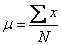where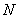is the number of scores. Mode: Mode of a set of data is the value in the set that occurs most often. Range: The range of a set of data is the difference between the highest and lowest values in the set. Variance: The variance of a sample measures how the conservations are spread around the mean. Standard Deviation: The standard deviation is the square root of the average of the squared deviations from the mean (variance). Categorical frequency distributions: Categorical frequency distributions can be used for data that can be placed in specific categories, such as nominal- or ordinal-level data. Central Limit Theorem: As the sample size increases the sampling distribution of the sample mean approaches the normal distribution with mean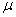and variance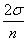.  The exponential, binomial, and normal density functions: The exponential, binomial and normal density functions are used to approximate statistical models and values.

Rapid Study Kit for "Title":
 Flash Movie Flash Game Flash Card Core Concept Tutorial Problem Solving Drill Review Cheat Sheet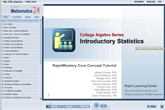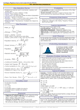"Title" Tutorial Summary : This tutorial mentions statistics and its different applications. Problem solving methods for statistics are mentioned in the examples. Knowing the data and its different aspects are important to the understanding of histograms and bar charts in statistics. The measures of central tendency are exhibited with the use of graphical representation. The measures of variability, range, deviation and standard deviation are important principles that are used in statistical problems. Hypothesis testing and the different types of error are presented in this tutorial. The Central Limit Theorem is defined and how it is applied to the different types of probability distributions.

 Tutorial Features: Specific Tutorial Features: • Examples showing the calculation of the mean, mode and variance are presented. • Hypothesis testing is presented with different alternatives and the use of examples. Series Features: • Concept map showing inter-connections of new concepts in this tutorial and those previously introduced. • Definition slides introduce terms as they are needed. • Visual representation of concepts • Animated examples—worked out step by step • A concise summary is given at the conclusion of the tutorial.

 "Title" Topic List: `Basic concepts of statistics Problem solving methods Measures of central tendency Mean Mode Midrange Range Variance Standard Deviation Random Variable Frequency Distribution and its graphStem-and-Leaf PlotProbability Distribution GraphTesting HypothesisAlternative and Null HypothesisCentral Limit Theorem`

See all 24 lessons in College Algebra, including concept tutorials, problem drills and cheat sheets: Teach Yourself College Algebra Visually in 24 Hours

 ©2014 Rapid Learning Center. Privacy Policy | Disclaimer Chemistry Survival Publishing, Biology Survival Publishing and Physics Survival Publishing are the divisions of Rapid Learning Inc.